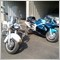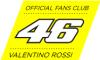# Indicators with alerts/signal - page 3594412

...

Those are crosses of 4 pairs of rsi : rsi_period1 over rsi_period2, rsi_period3 over rsi_period4, and so on ...

got it...thanks23

heiken ashi

hi,

i have been searching all over for a modification to give alerts crossing with the close of the candle with a moving average. would like to see arrows and have popups, sound, and email alert.below is the indicator that i use.....thanks for any help..........vince

Files:519

Anyone know if we have in Metatrader, this indicator:

?

this is the code for metastock:

var1 := (((2*C)-H)-L);

var1 := If(var1=0,0.01,var1);

var2 := (H-L);

var2 := If(var2=0,0.01,var2);

var3 := Sum((var1/var2)* VOLUME,21);

var4 := (Sum(VOLUME,21));

var5 := (var3/var4);

II := var5 *100;4412

...

bebeshel:
Anyone know if we have in Metatrader, this indicator:

?

this is the code for metastock:

var1 := (((2*C)-H)-L);

var1 := If(var1=0,0.01,var1);

var2 := (H-L);

var2 := If(var2=0,0.01,var2);

var3 := Sum((var1/var2)* VOLUME,21);

var4 := (Sum(VOLUME,21));

var5 := (var3/var4);

II := var5 *100;

"A common way to understand what has happened during a trading day is to calculate the Balance of Market Power, BMP. BMP consists of the close minus the opening divided by the high minus the low: which some call intraday intensity".

- "A common way to understand what has happened during a trading day"?????!!!! What has HAPPENED during a trading day???!!!!...Isn't it TOO LATE???:)259

Fibo indi with alarm?

--37

request for resistence and support indi

--350

--158

--350

--10